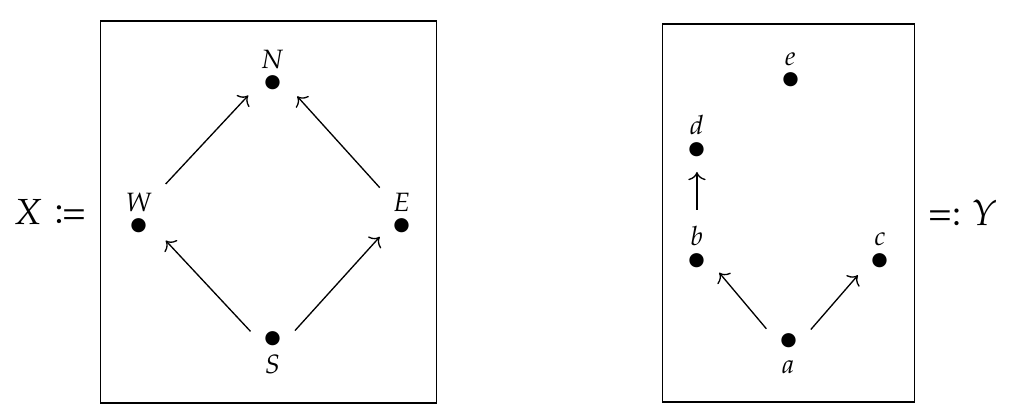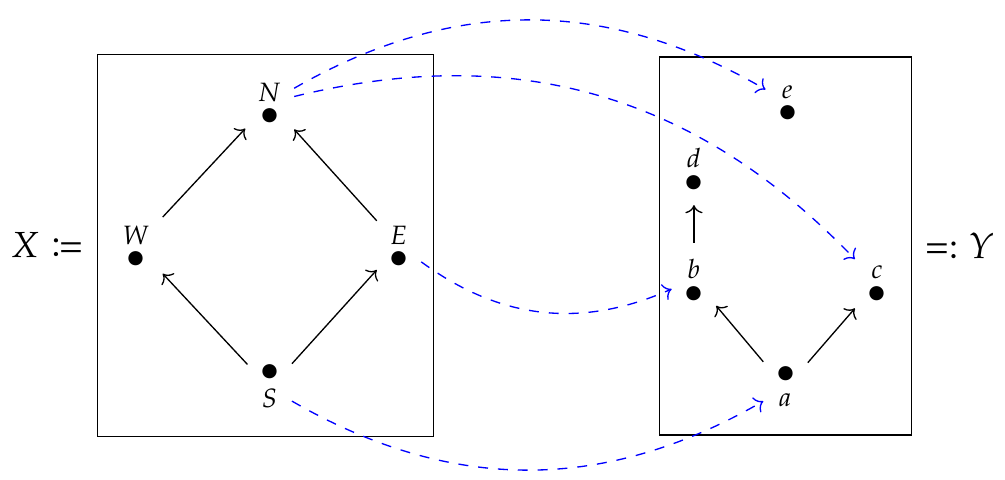Let's look at some examples of feasibility relations!

Feasibility relations work between preorders, but for simplicity suppose we have two posets \$$X\$$ and \$$Y\$$. We can draw them using [Hasse diagrams](https://en.wikipedia.org/wiki/Hasse_diagram):Here an arrow means that one element is less than or equal to another: for example, the arrow \$$S \to W\$$ means that \$$S \le W\$$. But we don't bother to draw all possible inequalities as arrows, just the bare minimum. For example, obviously \$$S \le S\$$ by reflexivity, but we don't bother to draw arrows from each element to itself. Also \$$S \le N\$$ follows from \$$S \le E\$$ and \$$E \le N\$$ by transitivity, but we don't bother to draw arrows that follow from others using transitivity. This reduces clutter.

(Usually in a Hasse diagram we draw bigger elements near the top, but notice that \$$e \in Y\$$ is not bigger than the other elements of \$$Y\$$. In fact it's neither \$$\ge\$$ or \$$\le\$$ any other elements of \$$Y\$$ - it's just floating in space all by itself. That's perfectly allowed in a poset.)

Now, we saw that a **feasibility relation** from \$$X\$$ to \$$Y\$$ is a special sort of relation from \$$X\$$ to \$$Y\$$. We can think of a relation from \$$X\$$ to \$$Y\$$ as a function \$$\Phi\$$ for which \$$\Phi(x,y)\$$ is either \$$\text{true}\$$ or \$$\text{false}\$$ for each pair of elements \$$x \in X, y \in Y\$$. Then a **feasibility relation** is a relation such that:

1. If \$$\Phi(x,y) = \text{true}\$$ and \$$x' \le x\$$ then \$$\Phi(x',y) = \text{true}\$$.

2. If \$$\Phi(x,y) = \text{true}\$$ and \$$y \le y'\$$ then \$$\Phi(x,y') = \text{true}\$$.

Fong and Spivak have a cute trick for drawing feasibility relations: when they draw a blue dashed arrow from \$$x \in X\$$ to \$$y \in Y\$$ it means \$$\Phi(x,y) = \text{true}\$$. But again, they leave out blue dashed arrows that would follow from rules 1 and 2, to reduce clutter!

Let's do an example:So, we see \$$\Phi(E,b) = \text{true}\$$. But we can use the two rules to draw further conclusions from this:

* Since \$$\Phi(E,b) = \text{true}\$$ and \$$S \le E\$$ then \$$\Phi(S,b) = \text{true}\$$, by rule 1.

* Since \$$\Phi(S,b) = \text{true}\$$ and \$$b \le d\$$ then \$$\Phi(S,d) = \text{true}\$$, by rule 2.

and so on.

**Puzzle 171.** Is \$$\Phi(E,c) = \text{true}\$$ ?

**Puzzle 172.** Is \$$\Phi(E,e) = \text{true}\$$?

I hope you get the idea! We can think of the arrows in our Hasse diagrams as _one-way streets_ going between cities in two countries, \$$X\$$ and \$$Y\$$. And we can think of the blue dashed arrows as _one-way plane flights_ from cities in \$$X\$$ to cities in \$$Y\$$. Then \$$\Phi(x,y) = \text{true}\$$ if we can get from \$$x \in X\$$ to \$$y \in Y\$$ _using any combination of streets and plane flights!_

That's one reason \$$\Phi\$$ is called a feasibility relation.

What's cool is that rules 1 and 2 can also be expressed by saying

$\Phi : X^{\text{op}} \times Y \to \mathbf{Bool}$

is a monotone function. And it's especially cool that we need the '\$$\text{op}\$$' over the \$$X\$$. Make sure you understand that: the \$$\text{op}\$$ over the \$$X\$$ but not the \$$Y\$$ is why we can drive _to_ an airport in \$$X\$$, then take a plane, then drive _from_ an airport in \$$Y\$$.

Here are some ways to lots of feasibility relations. Suppose \$$X\$$ and \$$Y\$$ are preorders.

**Puzzle 173.** Suppose \$$f : X \to Y \$$ is a monotone function from \$$X\$$ to \$$Y\$$. Prove that there is a feasibility relation \$$\Phi\$$ from \$$X\$$ to \$$Y\$$ given by

$\Phi(x,y) \text{ if and only if } f(x) \le y .$

**Puzzle 174.** Suppose \$$g: Y \to X \$$ is a monotone function from \$$Y\$$ to \$$X\$$. Prove that there is a feasibility relation \$$\Psi\$$ from \$$X\$$ to \$$Y\$$ given by

$\Psi(x,y) \text{ if and only if } x \le g(y) .$

**Puzzle 175.** Suppose \$$f : X \to Y\$$ and \$$g : Y \to X\$$ are monotone functions, and use them to build feasibility relations \$$\Phi\$$ and \$$\Psi\$$ as in the previous two puzzles. When is

$\Phi = \Psi ?$

**[To read other lectures go here.](http://www.azimuthproject.org/azimuth/show/Applied+Category+Theory#Chapter_4)**